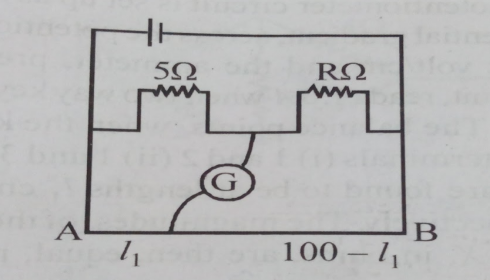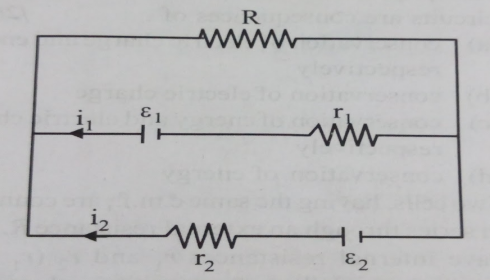## Filters

Sort by :
Clear All
Q
Medical
128 Views   |

Two cities are 150km apart. Electric power is sent from one city to another city through copper wires. the fall of potential per km is 8 volt and the average resistance per km is 0.5. The power loss in the wires is:

• Option 1)

19.2W

• Option 2)

19.2kW

• Option 3)

19.2J

• Option 4)

12.2kW

Power dissipiated in external resistance - -    Power loss= Power loss=  Option 1) 19.2W This option is incorrect. Option 2) 19.2kW This option is correct. Option 3) 19.2J This option is incorrect. Option 4) 12.2kW This option is incorrect.
Medical
94 Views   |

The resistance in the two arms of the meter bridge  are 5 and R, respectivilty. When the resistance R is shunted with an equal resistance, the new balance point is at . The resistance 'R' is:• Option 1)

10

• Option 2)

15

• Option 3)

20

• Option 4)

25

Meter bridge - To find the resistance of a given wire using a meter bridge and hence determine the specific resistance of its materials - wherein       Divide  and  Or,  From equation  Option 1) 10 This option is incorrect. Option 2) 15 This option is correct. Option 3) 20 This option is incorrect. Option 4) 25 This option is incorrect.
Medical
122 Views   |

See the electric circuit shown in the figure.Which of the following equations is a correct equation for it?

• Option 1)

• Option 2)

• Option 3)

• Option 4)

In closed loop - - wherein    Apply Kirchoff law: Option 1) This option is incorrect. Option 2) This option is incorrect. Option 3) This option is incorrect. Option 4) This option is correct.
Medical
111 Views   |

A potentiometer circuit has been set up for finding the internal resistance of a given cell. The main battery used across the potentiometer wire , has an emf of 2.0V and a negligible internal resistance. The potentiometer wire itself is 4m long, when the resistance R, connected across the given cell, has value of

(i) infinity              (ii) 9.5

The balancing lengths', on the potentiometer  wire are found to be 3 m and 2.85m, respectivily. The value of internal resistance of the cell is

• Option 1)

0.25

• Option 2)

0.95

• Option 3)

0.5

• Option 4)

0.75

Determine the internal resistance - - wherein    potential across wire =2V   => r= Option 1) 0.25 This is incorrect option  Option 2) 0.95 This is incorrect option  Option 3) 0.5 This is correct option  Option 4) 0.75 This is incorrect option